##### Statistics For Dummies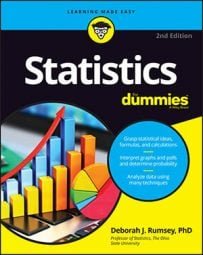You can compare numerical data for two statistical populations or groups (such as cholesterol levels in men versus women, or income levels for high school versus college grads) to test a claim about the difference in their averages. (For example, is the difference in the population means equal to zero, indicating their means are equal?) Two independent (totally separate) random samples need to be selected, one from each population, in order to collect the data needed for this test.

The null hypothesis is that the two population means are the same; in other words, that their difference is equal to 0. The notation for the null hypothesis is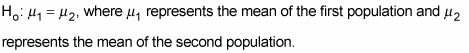You can also write the null hypothesis as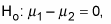emphasizing the idea that their difference is equal to zero if the means are the same.

The formula for the test statistic comparing two means (under certain conditions) is: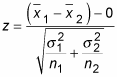To calculate it, do the following:

1. Calculate the sample means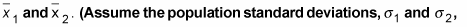are given.) Let n1 and n2 represent the two sample sizes (they need not be equal).

2. Find the difference between the two sample means: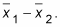Keep in mind that because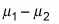is equal to 0 if H0 is true, it doesn’t need to be included in the numerator of the test statistic. However, if the difference they are testing is any value other than 0, you subtract that value from x1-x2 in the numerator of the test statistic.

3. Calculate the standard error using the following equation: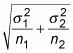4. Divide your result from Step 2 by your result from Step 3.

To interpret the test statistic, add the following two steps to the list:

5. Look up your test statistic on the standard normal (Z-) distribution (see the below Z-table) and calculate the p-value.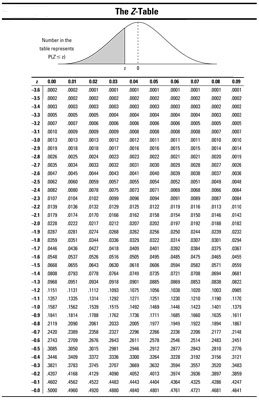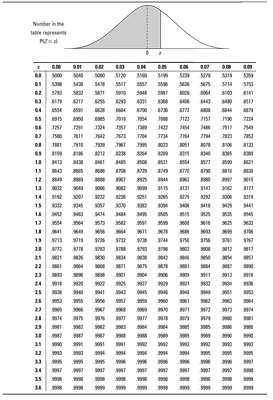6. Compare the p-value to your significance level, (such as 0.05). If it’s less than or equal to your significance level, reject H0. Otherwise, fail to reject H0.

The conditions for using this test are that the two population standard deviations are known and either both populations have a normal distribution or both sample sizes are large enough for the Central Limit Theorem to be applied.

For example, suppose you want to compare the absorbency of two brands of paper towels (call the brands Stats-absorbent and Sponge-o-matic). You can make this comparison by looking at the average number of ounces each brand can absorb before being saturated. H0 says the difference between the average absorbencies is 0 (nonexistent), and Ha says the difference is not 0. In other words, one brand is more absorbent than the other. Using statistical notation, you have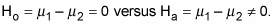Here, you have no indication of which paper towel may be more absorbent, so the not-equal-to alternative is the one to use.

Suppose you select a random sample of 50 paper towels from each brand and measure the absorbency of each paper towel. Suppose the average absorbency of Stats-absorbent (x1) for your sample is 3 ounces, and assume the population standard deviation is 0.9 ounces. For Sponge-o-matic (x2), the average absorbency is 3.5 ounces according to your sample; assume the population standard deviation is 1.2 ounces. Carry out this hypothesis test by following the 6 steps listed above:

1. Given the above information, you know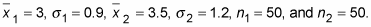2. The difference between the sample means for (Stats-absorbent – Sponge-o-matic) is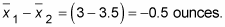(A negative difference simply means that the second sample mean was larger than the first.)

3. The standard error is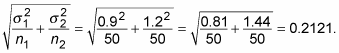4. Divide the difference, –0.5, by the standard error, 0.2121, which gives you –2.36. This is your test statistic.

5. To find the p-value, look up –2.36 on the standard normal (Z-) distribution — see the above Z-table. The chance of being beyond, in this case to the left of, –2.36 is equal to 0.0091. Because Ha is a not-equal-to alternative, you double this percentage to get 2 ∗ 0.0091 = 0.0182, your p-value.

6. This p-value is quite a bit less than 0.05. That means you have fairly strong evidence to reject H0.

Your conclusion is that a statistically significant difference exists between the absorbency levels of these two brands of paper towels, based on your samples. And Sponge-o-matic comes out on top, because it has a higher average. (Stats-absorbent minus Sponge-o-matic being negative means Sponge-o-matic had the higher value.)

The temptation is to say, “Well, I knew the claim that the absorbency levels were equal was wrong because one brand had a sample mean of 3.5 ounces and the other was 3.0 ounces. Why do I even need a hypothesis test?” All those numbers tell you is something about those 100 paper towels sampled. You also need to factor in variation using the standard error and the normal distribution to be able to say something about the entire population of paper towels.